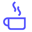a concise shot of dev knowledge
Become a Contributor
Concise shots of dev knowledge

RELATED TAGS

data mining

# What is the F1-score?Edpresso Team

The F1-score combines the precision and recall of a classifier into a single metric by taking their harmonic mean. It is primarily used to compare the performance of two classifiers. Suppose that classifier A has a higher recall, and classifier B has higher precision. In this case, the F1-scores for both the classifiers can be used to determine which one produces better results.

The F1-score of a classification model is calculated as follows:

$\frac{2(P * R)}{P+R}$

$P$ = the precision

$R$ = the recall of the classification model

## Example

### F1-score for a binary classifier

Consider the following confusion matrix that corresponds to a binary classifier:As computed earlier, the precision of the classifier equals $76.47 \%$, and the recall equals $81.25\%$. From these values, we can caluclate that the F1-score equals:

$\frac{2(76.47\% * 81.25\%)}{76.47\% + 81.25\%} = 78.79\%$

### F1-score for a multi-class classifier

Assume that we have calculated the following values:The F1-score for:

class A

$\frac{2(84\% * 80\%)}{84\%+80\%} = 81.95 \%$

class B

$\frac{2(79\% * 80\%)}{79\%+80\%} = 79.49 \%$

class C

$\frac{2(69\% * 73\%)}{69\%+73\%} = 70.94 \%$

From the calculations above, we can see that the classifier works best for class A.

One way to calculate the F1-score for the entire model is to take the arithmetic mean of the F1-scores of all the classes.

RELATED TAGS

data mining
RELATED COURSES

View all Courses

Keep Exploring

Learn in-demand tech skills in half the time